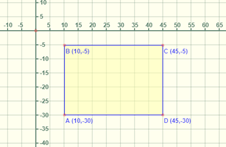Mathematics
Easy

Question

# Find the area of the rectangle whose coordinates are as follows## 110 sq. units    120 sq. units    825 sq. units    875 sq. unitsHint:

## The correct answer is: 875 sq. units

### The given rectangle ABCD is in fourth quadrant.The coordinates of the points are:A is (10,-30)B is (10,-5)C is (45,-5)D is (45,-30)We have to find the length of the adjacent sides.To find the length we will use the following criteria:1) If x coordinate is same, we will find the absolute distance using y coordinate. Absolute distance is its length from the respective axis. If x  coordinate is same, then  we will measure the length from x axis. It is a positive quantity.2) If y coordinate is same we will use the x coordinate to find absolute distance.3) As the points are in same quadrant, to find the length we will have to subtract the absolute distance.Let’s consider the side AB as length and BC as breadthWe will find the length of side AB.For the points A and B, x coordinate is same. We will find the absolute distance using y coordinate. It is distance from x axis.Absolute distance of the point A = |-30|Absolute distance of the point B = |-5|Length of AB = |-30| - |-5|= 30 – 5= 25Length of the rectangle is 25 units.We will find the length of side BC.For the points B and C, y coordinate is same. We will find the absolute distance using x coordinate. It is distance from y axis.Absolute distance of the point B = |-10|Absolute distance of the point C = |45|Length of BC = |45| - |-10|= 45 – 1= 35Breadth of the rectangle is 35 units.The formula for area is (length × breadth)Area of rectangle ABCD = (length × breadth)= (25 × 35)= 825 sq. unitsTherefore, area of the rectangle is 825 sq. units.

For such questions, we have to see if the points of the rectangle are in same quadrant or adjacent quadrants. For points in adjacent quadrants, we have to add the absolute values. We can use distance formula to find the length of sides of rectangle too.

### Related Questions to study#### With Turito Foundation.#### Get an Expert Advice From Turito.